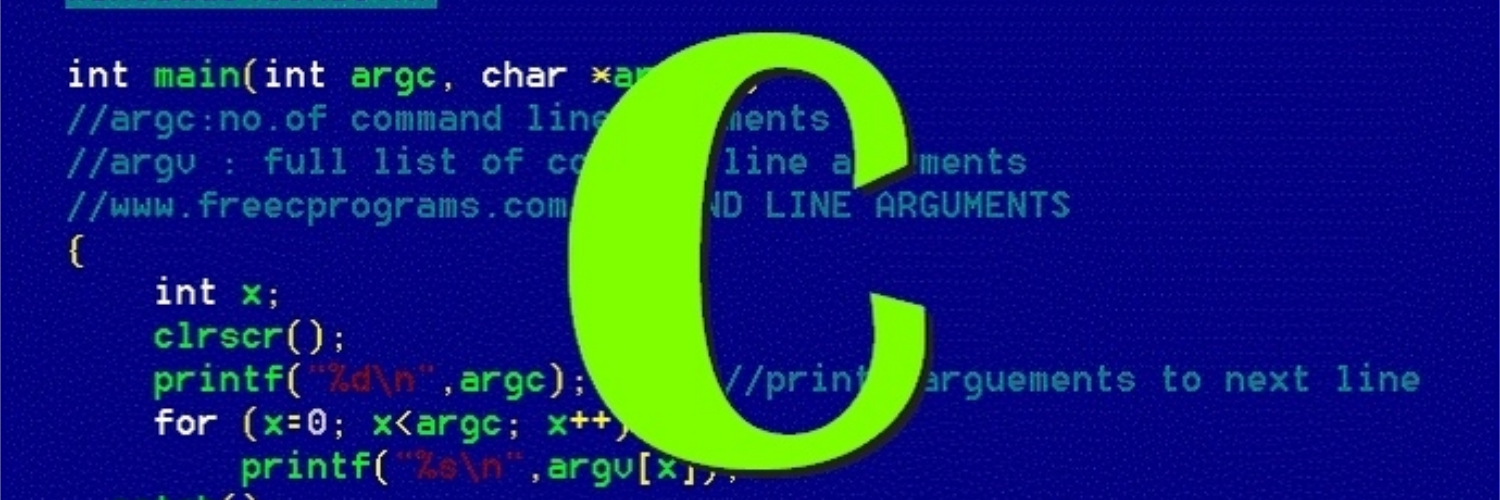# Practical C - Linear Search

Implement linear search in CI start this blog series to show some of the trickier parts of C programming.

In the first post, let’s implement the linear search in C.

# Integer Version

Let’s imagine that we search on an integer array.

Two things to notice here:

1. The function needs the array size n as a separate parameter.
2. Only the array’s address is passed in the function.

# Generic Basic Version

Let’s write a more generic version that does not specify type.

Here actually begins the interesting part of C: pointer manipulation.

First of all, in C there is no generics or templates like in Java or C++, the only solution is to use void *, which means a pointer to no matter what.

Let’s look at the function’s parameters.

1. void *key: a pointer to the key.
2. void *base: the array.
3. n: size of the array.
4. elemSize: the number of bytes each element in the array has.

Try to form the picture below in your mind.

The most difficult part here is how to iterate over the array. Pointer arithmatic does not work because that void * has no information about size and type. So here we have to do a hack. First we cast the void *base to a char *. Since char is always 1 byte, so this makes base a pointer to a 1 bytechar. To move forward i elements, the base needs to move i * elemSize bytes.

To compare the key and the current element in array. We just compare the bit pattern in memory using memcmp function. There is a problem with this approach, it does not work with an array of pointers. For example an array of C strings.

# True Generic Version

To solve the above problem, we have to tweak the implementation a little bit.

The code can be super confusing if you don’t truly understand pointer in C.

But let’s tackle this little by little.

First, we can see that the main difference is that the lsearch function now takes a function pointer cmp. This is not unfamiliar if you come from other OOP language like Java. The body of the lsearch remains mostly the same, except that now it uses the cmp function to compare two pointers instead of directly comparing the memory bit pattern.

The tricky part lies in writing the compare functions themselves.

Let’s look at the simpler one first, IntCmp.

It casts the void pointers to int pointers. And then dereference them and minus one from the other. Pretty straightforward.

Now let’s take a look at the StrCmp which is supposed to compare two strings.

Be careful that the void pointers here needs to be casted to pointers to char *s. And then dereference them, to get char *s, and then use the strcmp in C library to compare two strings.

One way to test your understanding is to try to implement different versions of linear search by yourself again, in a non-IDE text editor. Keep doing it until you feel totally fluent and comfortable about it.